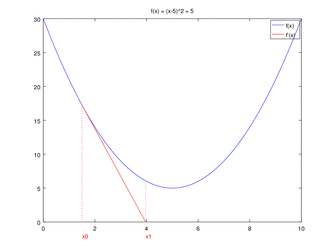# Newton raphson method for finding rootNewton Raphson Method

The Newton Raphson method is for solving equations of the form f(x) = 0. We make an initial guess for the root we are trying to find, and we call this initial guess x0.The sequence x0, x1, x2 ,x3, . . . generated in the manner described below should con-verge to the exact root.To implement it analytically we need a formula for each approximation in terms of the previous one, i.e. we need xn+1  in terms of xn.

The equation of the tangent line to the graph y = f(x) at the point (x0, f(x0)) isIts application to solving equations of the form f(x) = 0, as we now demonstrate, is called the Newton Raphson method.It is guaranteed to converge if the initial guess x0 is close enough, but it is hard to make a clear statement about what we mean by ‘close enough’ because this is highly problem specific. A sketch of the graph of f(x) can help us decide on an appropriate initial guess x0 for a particular problem.Nirajan Sah asked 4 months ago

Find the square root of 612 with an error less than 10-4 by newton-rapson method.Nirajan Sah answered 4 months ago

We have to find x such that

x2=612

The derivative of

f(x) = x2-612

is f’(x) = 2x

With an initial guess of x0=10, the sequence given by the method is

X1= x0 -(f(x0) /f'(x0)) = 10-(102-612)/(2*10)= 35.6

x2 = x1 - (f(x1)/f'(x1)) = 26.3955056

Similarly,

x3 = 24.7906355

x4 = 24.7386883

x5 = 24.7386338

Where the correct digits are underlined.

f(24.7386338) = (24.7386338)2-612 = 0.0000022905 <10-4

The required square root is 24.7386338.